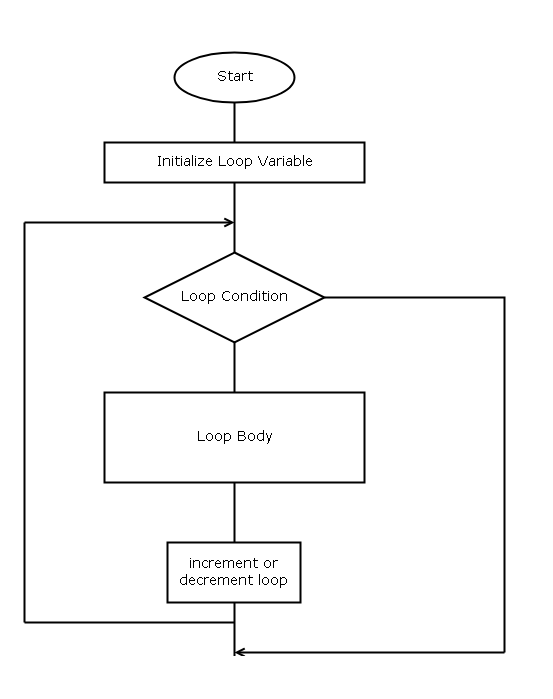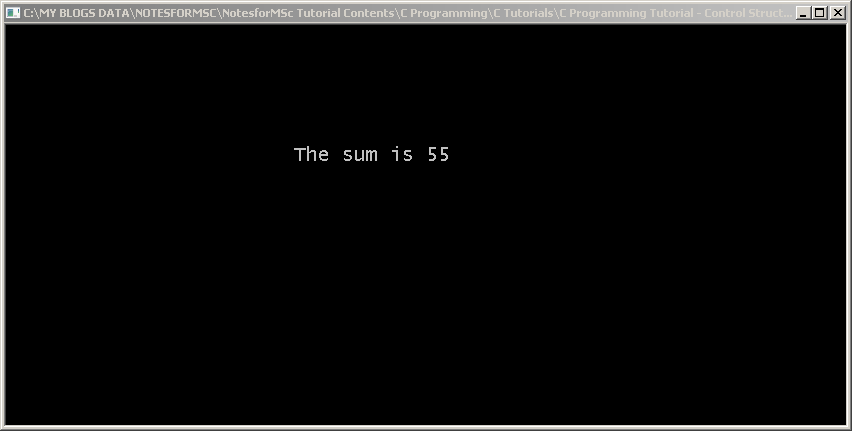## C Programming Tutorial – C While Loop

In C programming, we like to repeat some instructions over and over again with or without updated information. C language provides loops for this purpose.

The type of loop, you want to use depends on how you want to use the loop – C while loop, C for or C do-while loop.,/p>

• You may want to run the instruction for a fixed number of time.
• You may run the loop continuously until a specific condition terminates the loop.

C language provides 3 types of loop control structures.

• C While loop
• C For loop
• C Do-while loop

In the rest of the lesson, we will explore each loop structure in detail with examples and diagrams.

## C While Loop

The while loop is a simple loop which repeatedly execute statements within the body of loop as long as the condition to enter the loop is remains true. The while loop is terminated as soon as the condition becomes false.

### Parts of a while loop

1. Loop variable – a variable of type integer to keep tract of iterations of loop.
2. Loop condition – helps decide if loop body should be executed or not.
3. Loop body – task assigned within the loop that is performed repeatedly.

The steps performed during the execution of a while loop isDiagram – C while loop

Step 1: while loop is initialized by setting an initial value the loop variable.

Step 2: Condition for loop entry is checked against some value, if the output is true.

Step 3: The loop body is executed.

Step 4: loop variable is incremented for next iteration

Step 5: If the next iteration does not meet the loop condition and become false, loop body is not executed and terminates the while loop.

### Example Program – c while loop

``````#include <stdio.h>

int main()

{

int i, sum;

/* initialize the loop variable 'I' */

sum = 0;

i = 1;

/* set while loop condition */

while( i<=10)

{

sum = sum + i;

/* increment the loop */

i++;

}

/* print the result after loop terminates */

printf("\n\n\n\n\n\n");

printf("\t\t\tThe sum is %d\n",sum);

getch();

return 0;

}``````

In this example, we are going to repeatedly add numbers using a while loop and at the end print the results.

### Output – c while loopOutput – C while loop

### Example explained

The example program has a loop variable ‘I’ and we go through all the steps in the while loop execution and prints the output of the program after the loop terminates.

Step 1: The variable i and sum is initialized, where I is the loop variable.

``i = 1;``

Step 2: The variable I is tested in the loop condition and every time it is less than or equal to 10, the loop body is executed, otherwise it terminates the loop.

``while (i<=10)``

Step 3: If condition is true loop body is executed. The current value of I is added to the variable sum.

``sum = sum + i;``

Step 4: Increment the loop for next iteration.

``i++;``

Step 5: when the condition becomes false, i > 10, loop is terminated and output is printed to the console.

``printf(“\t\t\tThe sum is %d\n”,sum);``

### Common Errors

The common errors using while loop is as follows.

1. While loop not initialized properly.
2. Incorrect relational operator or value for conditions.
3. The condition must return a Boolean – true or false, otherwise loop does not work.
4. Loop variable not incremented or decremented.

### Bibliography

Balagurusamy, E. 2000. Programming in ANSI C. Tata McGraw-Hill Education,.

Kanetkar, Yashavant. 20 November 2002. Let us C. Bpb Publications.